# Why It’s So Hard to Surprise a Mathematician

Let’s be clear: it’s not so tough to startle a mathematician. I’ve made my wife jump simply by walking into a room (although, to my credit, I am stealthy like a spectacled fox).

But surprising a mathematician? That’s tough. And the reason why—well, it gets at the essence of what mathematicians do.Let’s begin with the Birthday “Paradox.” Note the skeptical quotation marks; it isn’t really a paradox. No laws of logic are harmed in the making of this film. It’s just a remarkable (and slightly eerie) surprise in elementary probability.

Scenario: People arrive, one by one, and add their birthdays to a big list at the front of the room. We keep going until a birthday is duplicated—i.e., until someone shows up whose birthday is already on the list.

How long does it take?

Answer: More often than not, you’ll be done after Person #23.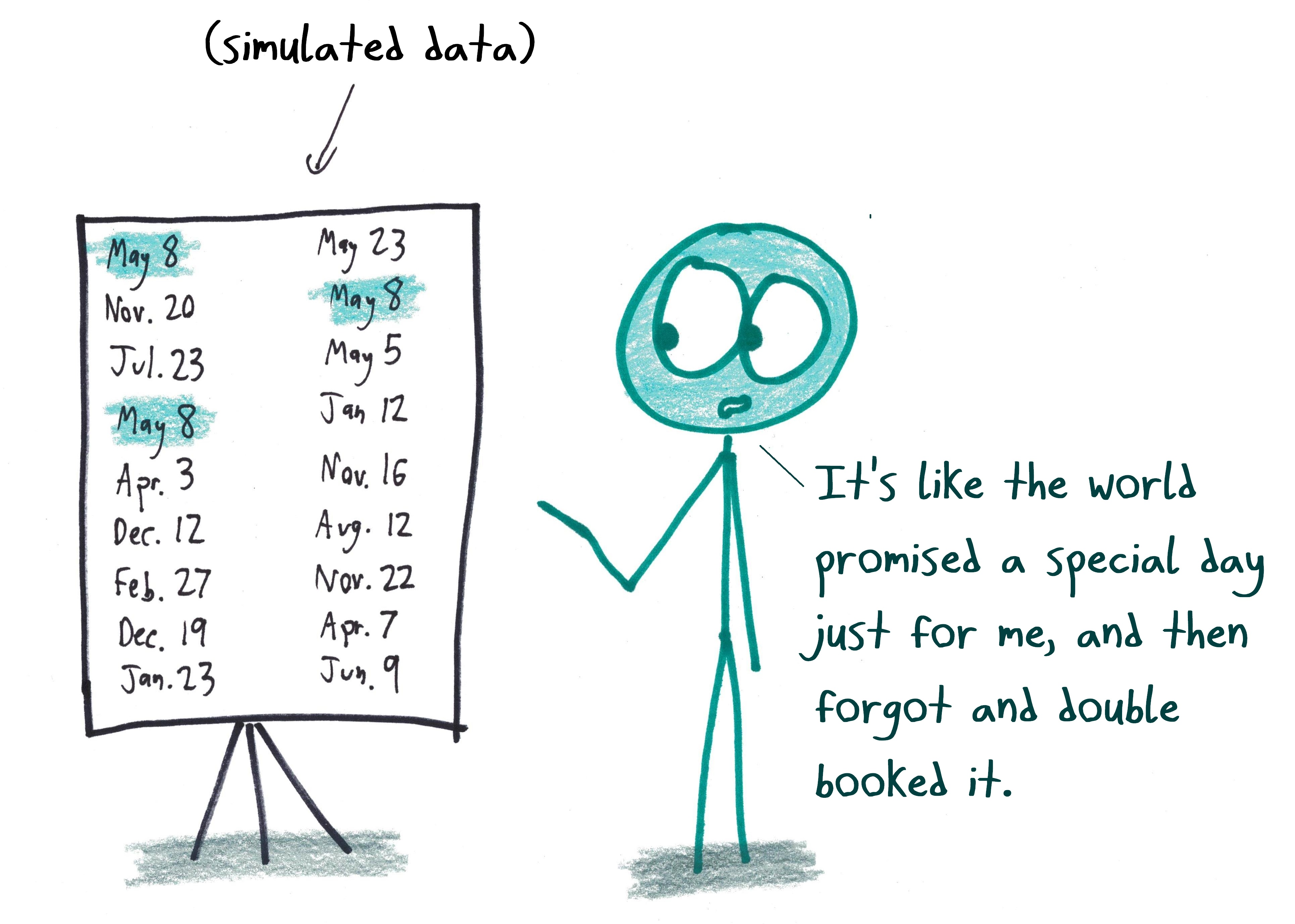When that fact first hit my brain, it left an impact crater. Only 23 people! The year has—last I checked—365 days, plus an extra one in Summer Olympics years. (Or the year just feels longer when there’s televised race-walking.) That only two dozen people suffice to produce a pair of Birthday Twins is an eye-popping surprise.

But here’s where things get paradoxical: the “birthday paradox” is so famous, so widely cited, that many math fans no longer find it surprising at all. They’ve internalized (even memorized) the number “23.” Hearing the fact again barely draws their attention.

How can you make this old tune sound new?

For that, I turn to my father, a man of tone-deaf musicality but perfect mathematical pitch, who showed me a nifty remix of the birthday paradox.

Scenario: Around the “Valley of a Thousand Tongues,” people speak 1000 mutually unintelligible languages. (Street signs here have very small print.) Every day, people gather at random in the valley, one by one, until two people speak the same language.

How many arrivals does it take before there’s a pair who can understand one another?

Answer: It’ll usually happen by the 38th person.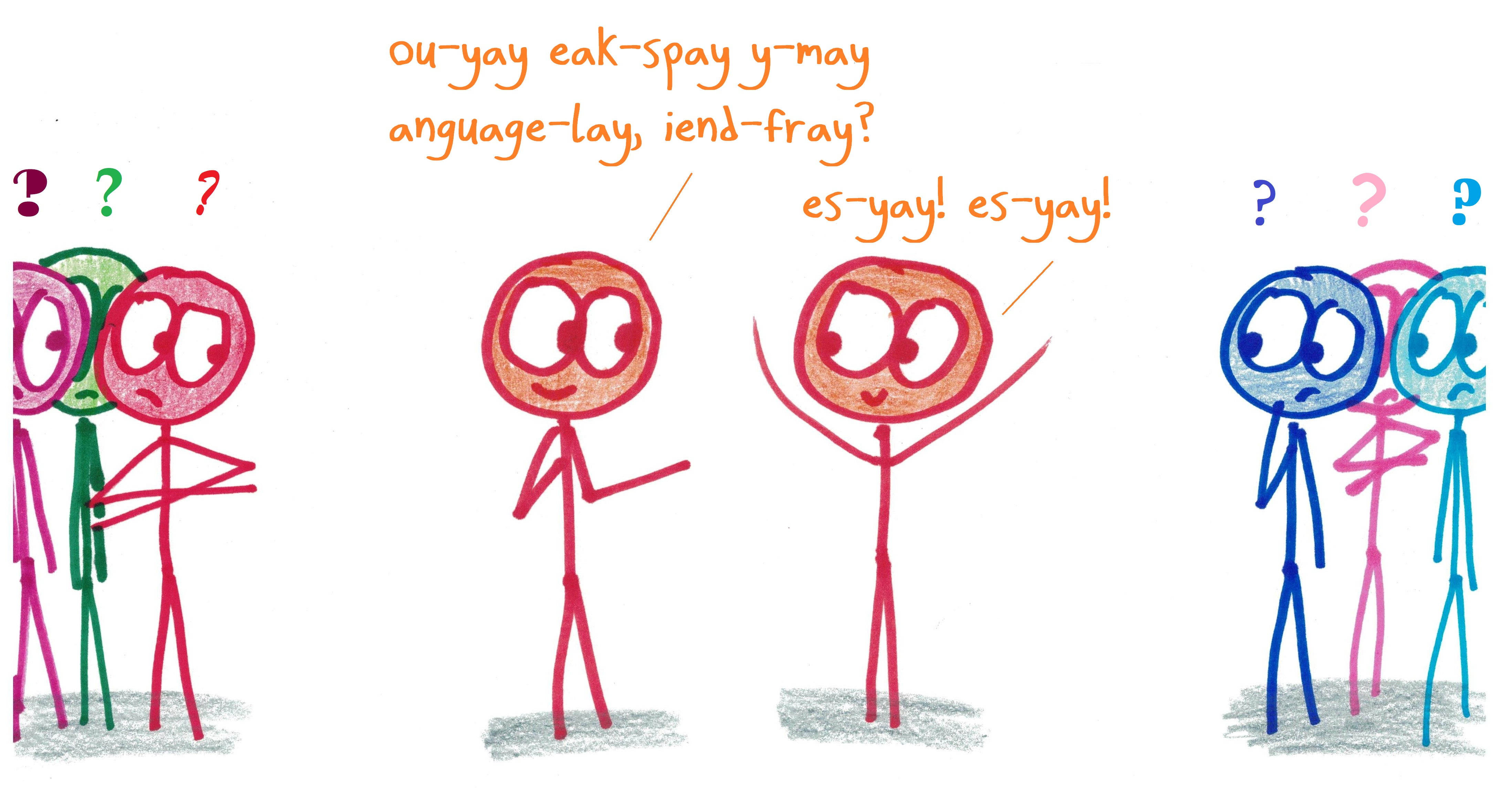This is, of course, just a slightly scaled-up birthday paradox. Roughly triple the number of possibilities (from 365 to 1000 ), and you only increase the number of people needed by about 50%.

And, going even further…

Scenario: Every US resident (there are ~320 million of us) gets a 9-digit social security number. That allows for a billion possible numbers. It’ll be generations before we start needing to recycle numbers – as long as we’re systematic about it.

But what if, instead, we just generated a random number for each person? How long before the random number generator produced a pair of duplicate numbers?

Answer: You’ll usually hit a duplicate in the first 40,000 people. That’s before you’re done with UCLA students.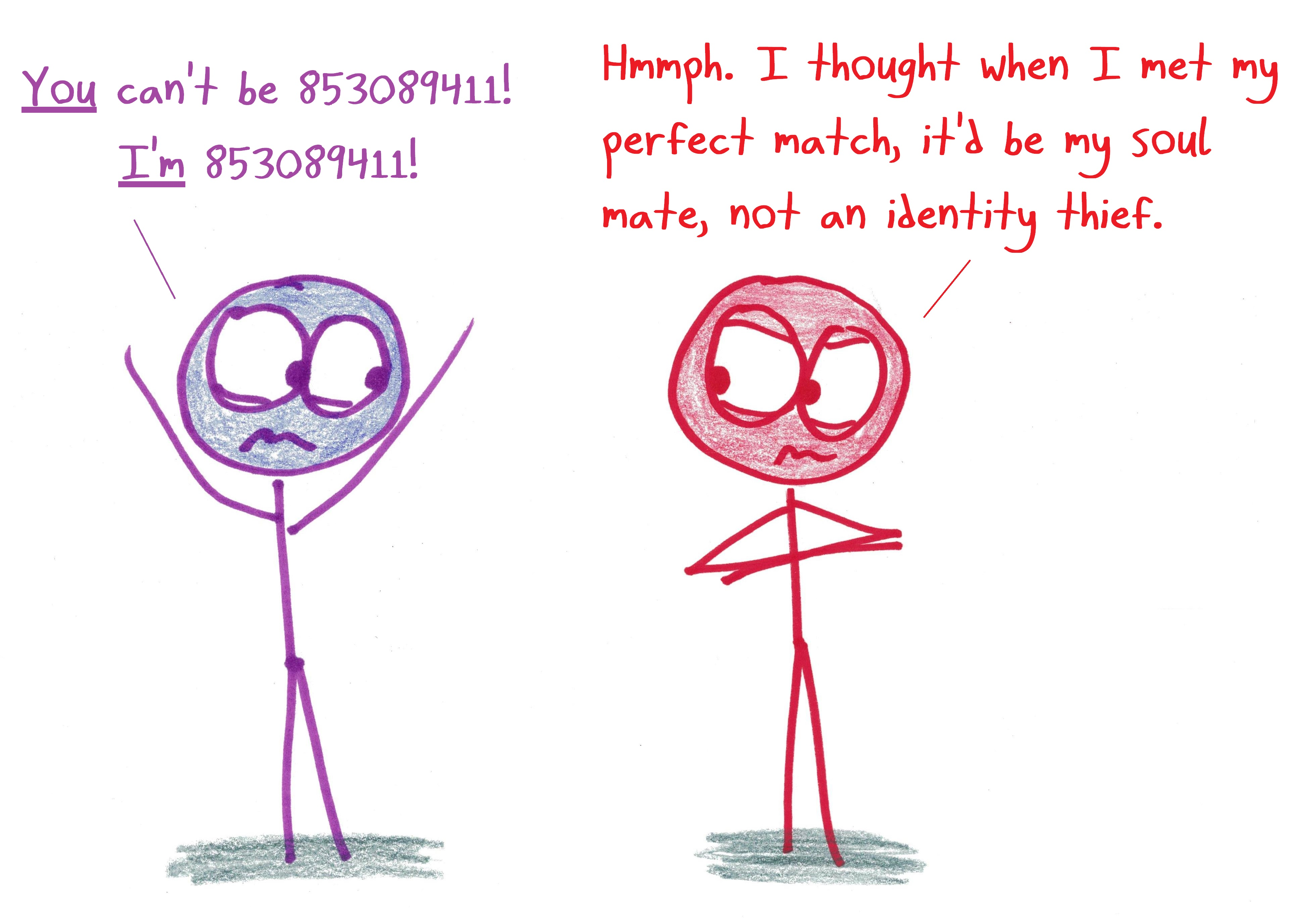Surprised?

Or is the surprise starting to fade?

This isn’t really about birthdays, dialects, or ID numbers. It’s about coincidences. What we’re seeing here is that coincidences occur all the time – much more than naive intuition might expect. And the mathematician has a natural question: WHY?

In each of these cases, we’re looking for lucky correspondences between pairs of people. And the essential fact is that even small groups have many pairs of people.

For example, in a room of just 10 folks, there are 45 possible pairs:Or, in a room of 23 people, there are an impressive 253 possible pairs. That’s enough to make it likelier than not that two will share the same birthday.In a room of 38 people, there are a whopping 703 possible pairs. That’s why, even with 1000 languages, you’re likely to find a pair speaking the same one.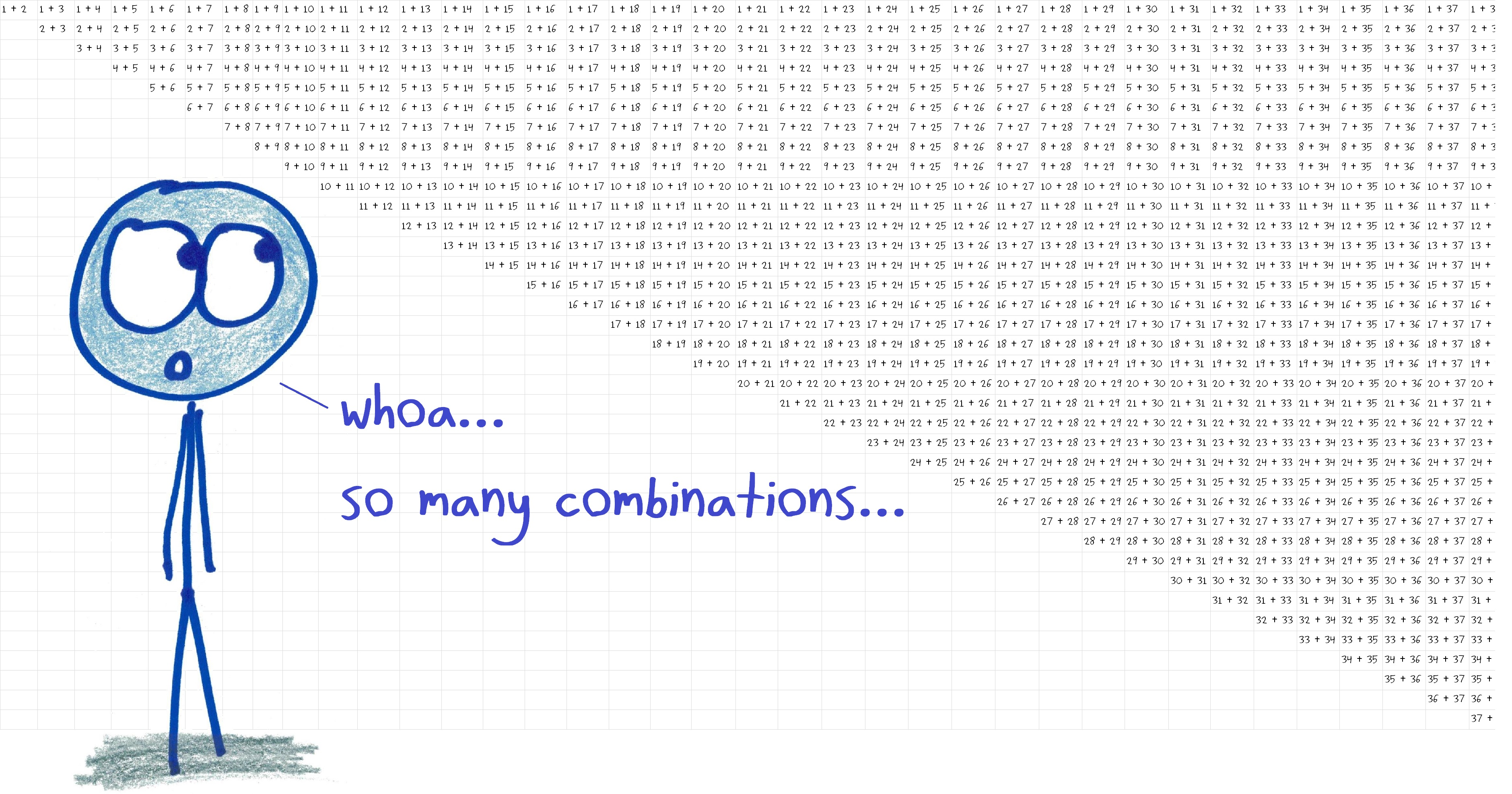Finally, in a room of 40,000 people, there are an incredible 800 million possible pairs. Even with a billion numbers to choose from, a random assignment will probably give the same number to at least two of them.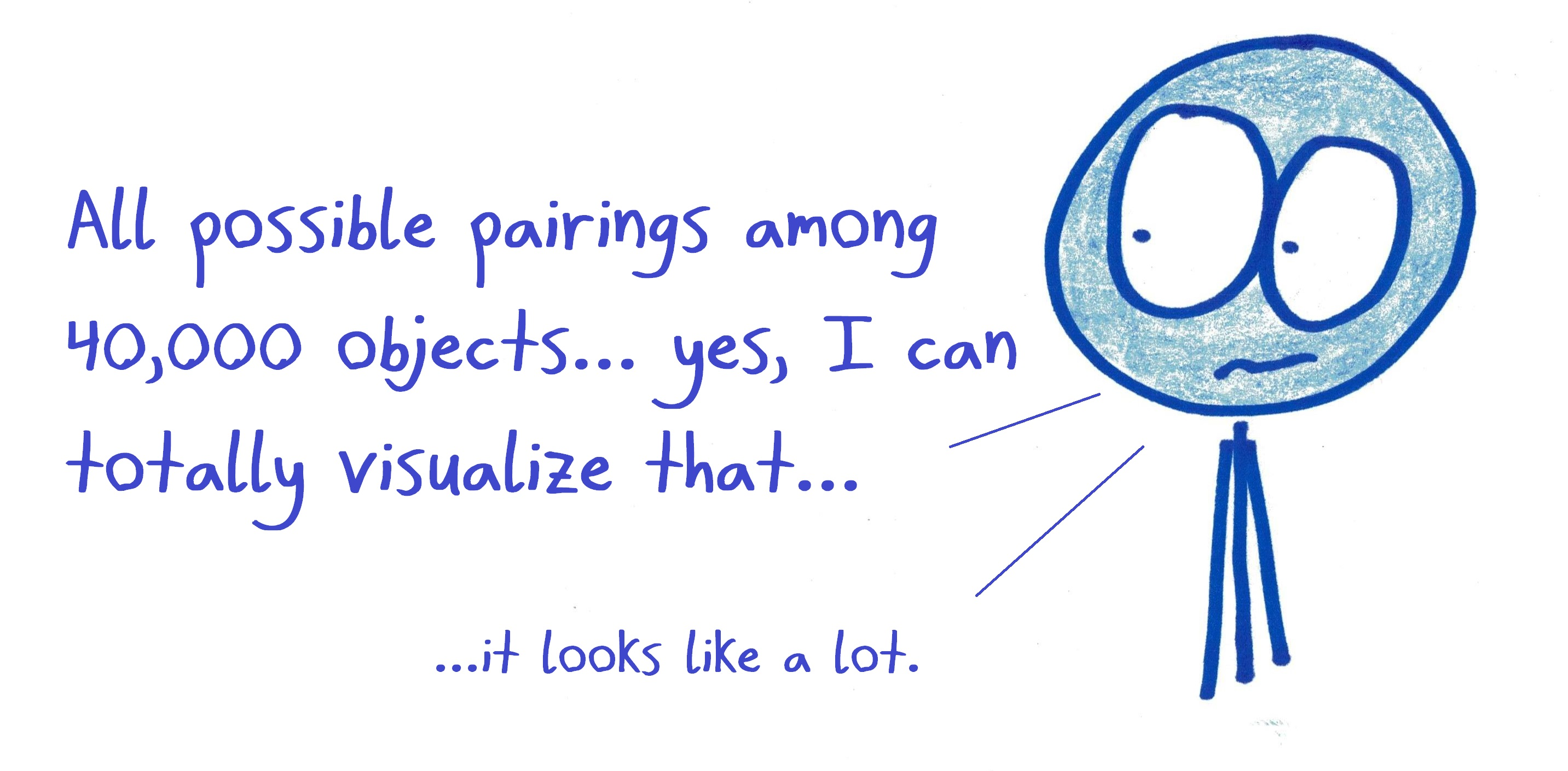Once you’ve explored these computations, a pattern emerges.  You can absorb all of these cases (and more) under the same heuristic, the same loose but powerful understanding: that as a group grows in size, the number of pairs within the group scales very fast.

And once you do this, you become awfully hard to surprise.

The world of mathematics is full of dazzling, wormhole-through-reality connections. Mathematicians spend their days seeking these out. And then, once they’ve found them, they do everything in their considerable power to neutralize the surprises, to reduce them to the simplest terms, to reinterpret them as the inevitable consequences of elementary assumptions.

Because of this, there’s a special joy and challenge in surprising a mathematician. You’ve got to find some strangeness, some peculiar truth, which is intrinsic to logic itself. That’s no easy task—but damn, is it satisfying.

## 15 thoughts on “Why It’s So Hard to Surprise a Mathematician”

1.Michael Paul Goldenberg says:

Hmm. I’m immediately tempted to mention “the unexpected hanging” paradox and variations thereupon (which I imagine you could come up with easily). I believe that even a condemned mathematician would be “surprised” when they come to hang her. I realize to some extent we’re “playing” with semantics, of course.

1.fred says:

According to Kripke, the “unexpected hanging” paradox is based on an actual civil defense exercise in Sweden, announced to be a surprise during a specified week. Quine turned that into a surprise hanging, Kripke into a surprise exam, which says more about both these guys than about the underlying logic

2.imagineambition says:

Maybe that’s why I’m “unexcitable” according to a loser I once dated. There isn’t much that can happen that surprises me in this world.

3.Aditya Chincholi says:

The surprise element is really about the$O(n^2)$ dependence of the number of pairs here. A more extreme version of this happened to me when I was learning about the orders of growth: linear, quadratic, logarithmic and exponential.

Say if a log algorithm takes 10 ns then
a linear one takes 1 microseconds,
a quadratic one takes 1 millisecond and
an exponential one would take$10^{284} yrs$!

1.Ben Orlin says:

Ha! That’s a great demonstration. I feel like I will never known enough about orders of growth to stop being surprised by things like that.

1.Michael Paul Goldenberg says:

“I will never known enough about orders of growth to stop being surprised by things like that.”

Which is surprising. ;^)

1.Ben Orlin says:

Ha – fair! The only unsurprising part of it is that I made the typo “known” instead of “know”…

4.Nazgand says:

Maybe generalize to finding the probability that at least k independent uniformly random variables fit the same profile with m profiles and n total people.

Take a moment to marvel at the fact that the number of possible triads is O(n^3), and the number of possible pairs is O(n^2), yet the existence of a triad implies a pair. I.e. P(O(n^3))<P(O(n^2)).
Take a moment to marvel at the fact that the number of possible quads is O(n^4), and the number of possible triads is O(n^3), yet the existence of a quad implies a triad. I.e. P(O(n^4))<P(O(n^3)).

This is no more startling than the previous `paradox'.
The problem is that the number of possible k-tuples does not directly translate to the probability of the existence of a monochrome k-tuple. P(monochrome k-tuple exists in group size n with m colors)=1 if (k-1)m<n.

Let the colors be the numbers$\{1,\dots,m\}$, respectively occurring with probabilities$\{p_1,\dots,p_m\}\subseteq\mathbb{R}$.$p_j\geq 0$ and$1\geq\sum_{j=1}^m p_j$
Let the set of things be size$n$.
The probability of exactly$k$ things having color$j$ is$p_j^k\left(1-p_j\right)^{n-k}$.
Thus, the probability of at least$c$ things having color$j$ is$\sum_{k=c}^n p_j^k\left(1-p_j\right)^{n-k} = 1 - \sum_{k=0}^c-1 p_j^k\left(1-p_j\right)^{n-k}$.
Thus, the probability of at least$c$ things having the same color is$1 - \prod_{j=1}^{m}\sum_{k=0}^{c-1} p_j^k\left(1-p_j\right)^{n-k}$.

1.Nazgand says:

Typo correction:
Thus, the probability of at least$c$ things having color$j$ is$\sum_{k=c}^n p_j^k\left(1-p_j\right)^{n-k} = 1 - \sum_{k=0}^{c-1} p_j^k\left(1-p_j\right)^{n-k}$.

2.Nazgand says:

I realized before I slept that I forgot the binomial coefficient. Correction:

Let the colors be the numbers$\{1,\dots,m\}$, respectively occurring with probabilities$\{p_1,\dots,p_m\}\subseteq\mathbb{R}$.$p_j\geq 0$ and$1\geq\sum_{j=1}^m p_j$
Let the set of things be size$n$.
The probability of exactly$k$ things having color$j$ is$\binom{n}{k} p_j^k\left(1-p_j\right)^{n-k}$.
Thus, the probability of at least$c$ things having color$j$ is$\sum_{k=c}^n \binom{n}{k} p_j^k\left(1-p_j\right)^{n-k} = 1 - \sum_{k=0}^{c-1} \binom{n}{k} p_j^k\left(1-p_j\right)^{n-k}$.

The last step involving the product is also incorrect because it ignores conditional probabilities.
I will look again later at the recursive method of solving the problem for insight.

5.Roslyn Miller says:

Thanks for your fresh perspective on the Birthday “Paradox!” As a lover of mathematics and people and bringing mathematics to people, I dig and can’t wait to use the expansion of the concept. I believe this is my first time to comment on your blog, but I always appreciate your combination of genius and productivity to enlighten and delight through your blog.

6.Nick Ryan says:

The Valley of a Thousand Tongues, which sounds horrifying now that I write it, made me curious about another problem which I cannot immediately figure out how to answer. What if the question wasn’t when one pair could communicate, but at what population should we expect every possible pair to be able to communicate based on connecting pairs of communicators?

I will continue to think about it in my spare time, but I feel like I may not know enough math to figure it out. I’d appreciate anyone who could point me in the right direction analytically.

1.holdenrhit says:

You’d have to change the set-up such that either some languages are mutually intelligible, or some people speak more than one language. In either case, my intuition is that it’s pretty quick to reach the situation where you expect *almost* everyone can communicate, and very slow (or possibly never?) to reach the point where you expect *everyone* can communicate. (I would model it with random graph theory and estimate the chances of having exactly one connected component. https://en.wikipedia.org/wiki/Random_graph might help you get started.)